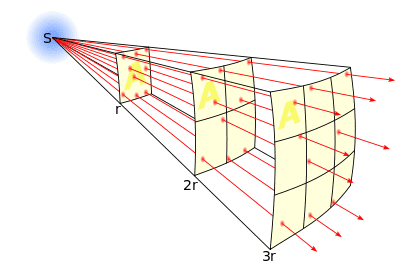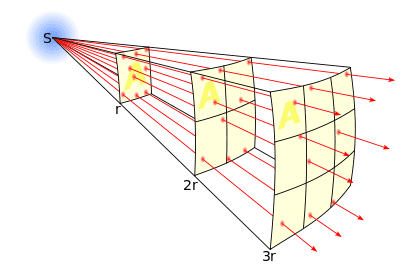# Who Created the Inverse Square Law Theory

• B
• InverseSquareLaw
The ISL is a mathematical relationship that describes how the force of gravity varies with distance. It was first postulated by Isaac Newton in 1687, and has been used to model a wide variety of physical phenomena.

#### InverseSquareLaw

I Am Wanting to Find out who Created the inverse square law.I'd say the first use of it was probably Isaac Newton, who discovered that the force of gravitation falls off in strength at a ratio of 1/r2, which is known as the inverse square law.

Drakkith said:
the force of gravitation falls off in strength at a ratio of 1/r2
It would have been much easier verify the ISL using light. His astronomical observations would not have given a direct 'proof' of ISL but he could have easily verified the effect of the ISL law with a simple photometer. You could do it yourself, on the 'kitchen table' with some light bulbs, tracing paper, oil and some cardboard boxes, painted black, to exclude extraneous light and reflections. No light meters need to be involved.
Here you are. That could be you and me in the front picture.(I thought, at first, the guy on the left was in his PJs.)

•Drakkith
InverseSquareLaw said:
I Am Wanting to Find out who Created the inverse square law.The ISL was known long before Newton came along, and not only in connection with gravitational concepts:

https://en.wikipedia.org/wiki/Inverse-square_law

The earliest indication from history is that the ISL could explain an observed physical phenomenon came from Oxford in the mid-14th century concerning the intensity of illumination. Later, the concept was picked up by other scientists, including Kepler, who suspected but could not prove that planets in orbit about the sun obeyed an ISL with respect to the force of attraction between them.

Other scientists in England, including Robert Hooke, Christopher Wren, and Edmond Halley, also subscribed to the idea of gravitation obeying the ISL, but it was Newton who showed that by calculation, the assumption of the ISL for gravity led directly to Kepler's laws of motion, and this astonishing result formed the basis for Halley to urge a reluctant Newton to write the Principia.

•Drakkith

## 1. Who is credited with creating the Inverse Square Law Theory?

The Inverse Square Law Theory was first formulated by the English physicist Isaac Newton in his book "Philosophiæ Naturalis Principia Mathematica" in 1687.

## 2. What is the Inverse Square Law Theory?

The Inverse Square Law Theory states that the intensity of an effect (such as light, sound, or gravitational force) is inversely proportional to the square of the distance from the source of that effect.

## 3. What evidence supports the Inverse Square Law Theory?

The Inverse Square Law Theory has been observed and tested in many scientific experiments, including the famous experiments by Henry Cavendish and Robert Millikan on gravitational and electric forces, respectively.

## 4. How does the Inverse Square Law Theory apply to different phenomena?

The Inverse Square Law Theory can be applied to a wide range of phenomena, including the brightness of light as it spreads out from a source, the loudness of sound as it travels through the air, and the strength of gravitational and electric forces as they act on objects at varying distances.

## 5. Is the Inverse Square Law Theory still considered valid today?

Yes, the Inverse Square Law Theory is still considered valid and is used in many fields of science, including physics, astronomy, and engineering. It is a fundamental principle that helps us understand and predict the behavior of natural phenomena.